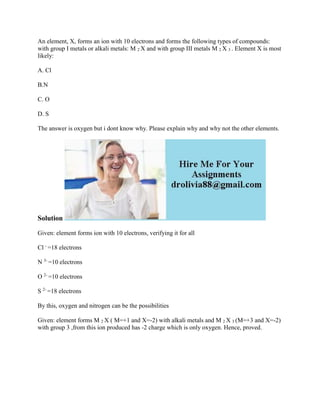Successfully reported this slideshow.

# An element- X- forms an ion with 10 electrons and forms the following.docx×

# An element- X- forms an ion with 10 electrons and forms the following.docx

An element, X, forms an ion with 10 electrons and forms the following types of compounds: with group I metals or alkali metals: M 2 X and with group III metals M 2 X 3 . Element X is most likely:
A. Cl
B.N
C. O
D. S
The answer is oxygen but i dont know why. Please explain why and why not the other elements.
Solution
Given: element forms ion with 10 electrons, verifying it for all
Cl - =18 electrons
N 3- =10 electrons
O 2- =10 electrons
S 2- =18 electrons
By this, oxygen and nitrogen can be the possibilities
Given: element forms M 2 X ( M=+1 and X=-2) with alkali metals and M 2 X 3 (M=+3 and X=-2) with group 3 ,from this ion produced has -2 charge which is only oxygen. Hence, proved.
.

An element, X, forms an ion with 10 electrons and forms the following types of compounds: with group I metals or alkali metals: M 2 X and with group III metals M 2 X 3 . Element X is most likely:
A. Cl
B.N
C. O
D. S
The answer is oxygen but i dont know why. Please explain why and why not the other elements.
Solution
Given: element forms ion with 10 electrons, verifying it for all
Cl - =18 electrons
N 3- =10 electrons
O 2- =10 electrons
S 2- =18 electrons
By this, oxygen and nitrogen can be the possibilities
Given: element forms M 2 X ( M=+1 and X=-2) with alkali metals and M 2 X 3 (M=+3 and X=-2) with group 3 ,from this ion produced has -2 charge which is only oxygen. Hence, proved.
.